# 3.1 Functions and function notation  (Page 9/21)

 Page 9 / 21

We will see these toolkit functions, combinations of toolkit functions, their graphs, and their transformations frequently throughout this book. It will be very helpful if we can recognize these toolkit functions and their features quickly by name, formula, graph, and basic table properties. The graphs and sample table values are included with each function shown in [link] .

Toolkit Functions
Name Function Graph
Constant $f\left(x\right)=c,\text{\hspace{0.17em}}$ where $c$ is a constant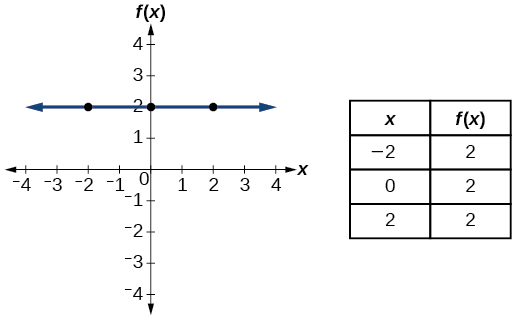Identity $f\left(x\right)=x$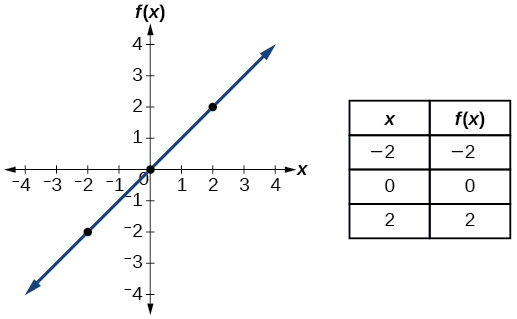Absolute value $f\left(x\right)=|x|$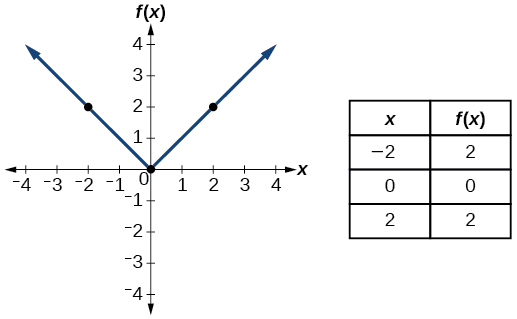Quadratic $f\left(x\right)={x}^{2}$Cubic $f\left(x\right)={x}^{3}$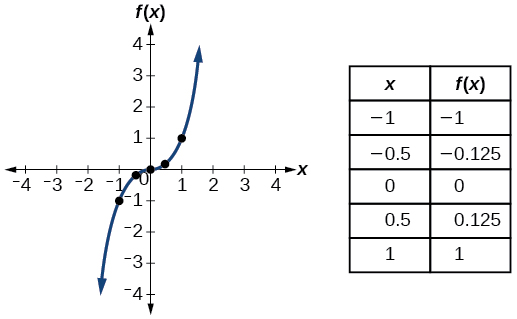Reciprocal $f\left(x\right)=\frac{1}{x}$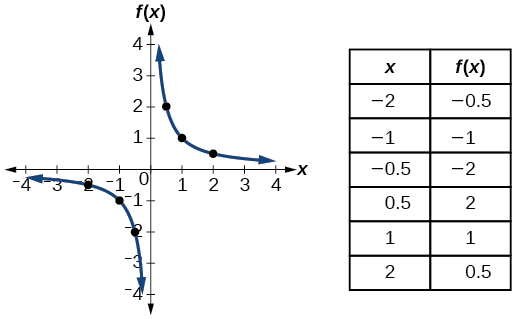Reciprocal squared $f\left(x\right)=\frac{1}{{x}^{2}}$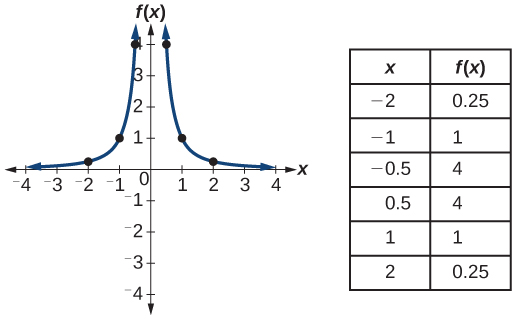Square root $f\left(x\right)=\sqrt{x}$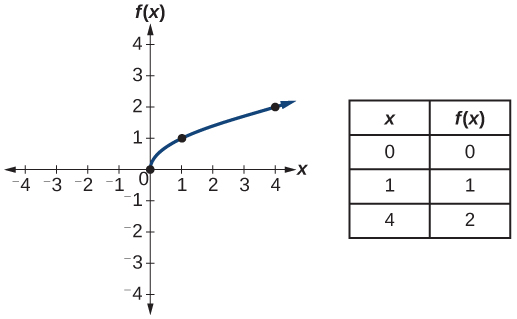Cube root $f\left(x\right)=\sqrt{x}$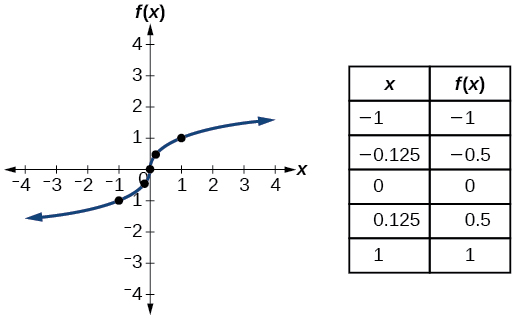Access the following online resources for additional instruction and practice with functions.

## Key equations

 Constant function $f\left(x\right)=c,$ where $\text{\hspace{0.17em}}c\text{\hspace{0.17em}}$ is a constant Identity function $f\left(x\right)=x$ Absolute value function $f\left(x\right)=|x|$ Quadratic function $f\left(x\right)={x}^{2}$ Cubic function $f\left(x\right)={x}^{3}$ Reciprocal function $f\left(x\right)=\frac{1}{x}$ Reciprocal squared function $f\left(x\right)=\frac{1}{{x}^{2}}$ Square root function $f\left(x\right)=\sqrt{x}$ Cube root function $f\left(x\right)=\sqrt{x}$

## Key concepts

• A relation is a set of ordered pairs. A function is a specific type of relation in which each domain value, or input, leads to exactly one range value, or output. See [link] and [link] .
• Function notation is a shorthand method for relating the input to the output in the form $\text{\hspace{0.17em}}y=f\left(x\right).\text{\hspace{0.17em}}$ See [link] and [link] .
• In tabular form, a function can be represented by rows or columns that relate to input and output values. See [link] .
• To evaluate a function, we determine an output value for a corresponding input value. Algebraic forms of a function can be evaluated by replacing the input variable with a given value. See [link] and [link] .
• To solve for a specific function value, we determine the input values that yield the specific output value. See [link] .
• An algebraic form of a function can be written from an equation. See [link] and [link] .
• Input and output values of a function can be identified from a table. See [link] .
• Relating input values to output values on a graph is another way to evaluate a function. See [link] .
• A function is one-to-one if each output value corresponds to only one input value. See [link] .
• A graph represents a function if any vertical line drawn on the graph intersects the graph at no more than one point. See [link] .
• The graph of a one-to-one function passes the horizontal line test. See [link] .

## Verbal

What is the difference between a relation and a function?

A relation is a set of ordered pairs. A function is a special kind of relation in which no two ordered pairs have the same first coordinate.

What is the difference between the input and the output of a function?

Why does the vertical line test tell us whether the graph of a relation represents a function?

When a vertical line intersects the graph of a relation more than once, that indicates that for that input there is more than one output. At any particular input value, there can be only one output if the relation is to be a function.

How can you determine if a relation is a one-to-one function?

#### Questions & Answers

write down the polynomial function with root 1/3,2,-3 with solution
if A and B are subspaces of V prove that (A+B)/B=A/(A-B)
write down the value of each of the following in surd form a)cos(-65°) b)sin(-180°)c)tan(225°)d)tan(135°)
Prove that (sinA/1-cosA - 1-cosA/sinA) (cosA/1-sinA - 1-sinA/cosA) = 4
what is the answer to dividing negative index
In a triangle ABC prove that. (b+c)cosA+(c+a)cosB+(a+b)cisC=a+b+c.
give me the waec 2019 questions
the polar co-ordinate of the point (-1, -1)
prove the identites sin x ( 1+ tan x )+ cos x ( 1+ cot x )= sec x + cosec x
tanh`(x-iy) =A+iB, find A and B
B=Ai-itan(hx-hiy)
Rukmini
what is the addition of 101011 with 101010
If those numbers are binary, it's 1010101. If they are base 10, it's 202021.
Jack
extra power 4 minus 5 x cube + 7 x square minus 5 x + 1 equal to zero
the gradient function of a curve is 2x+4 and the curve passes through point (1,4) find the equation of the curve
1+cos²A/cos²A=2cosec²A-1
test for convergence the series 1+x/2+2!/9x3ByByByBy Jordon Humphreys